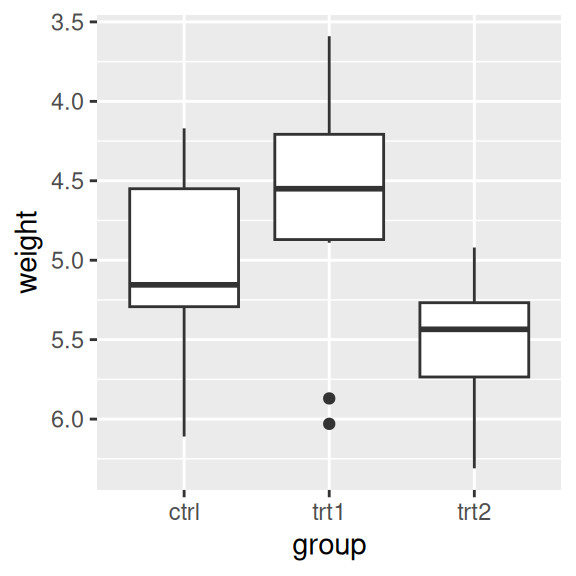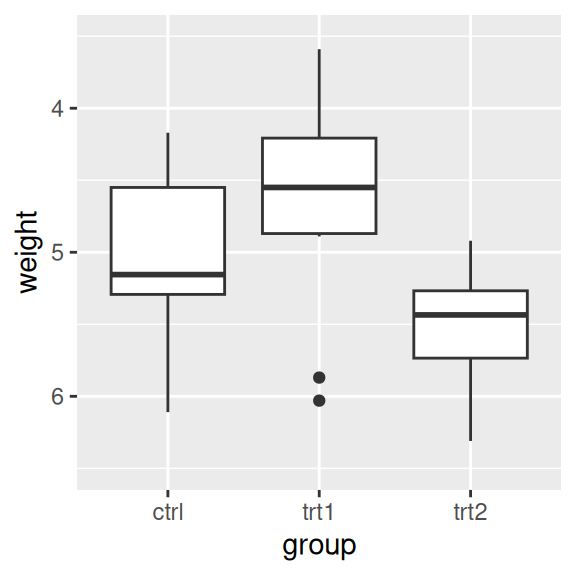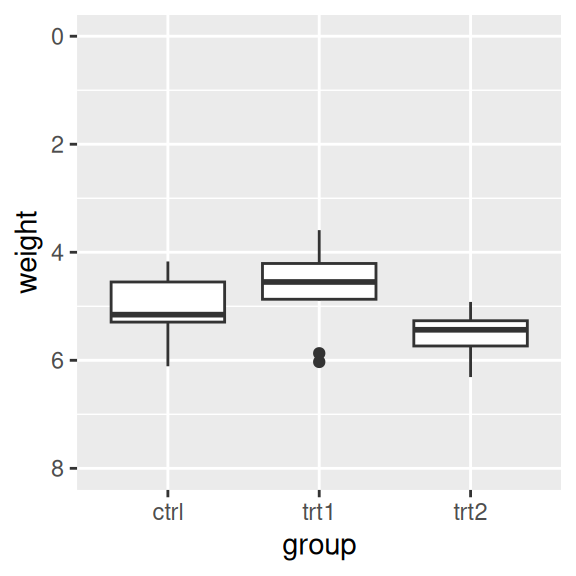## 8.3 Reversing a Continuous Axis

### 8.3.1 Problem

You want to reverse the direction of a continuous axis.

### 8.3.2 Solution

Use `scale_y_reverse()` or `scale_x_reverse()` (Figure 8.6). The direction of an axis can also be reversed by specifying the limits in reversed order, with the maximum first, then the minimum:

``````ggplot(PlantGrowth, aes(x = group, y = weight)) +
geom_boxplot() +
scale_y_reverse()

# Similar effect by specifying limits in reversed order
ggplot(PlantGrowth, aes(x = group, y = weight)) +
geom_boxplot() +
ylim(6.5, 3.5)``````Figure 8.6: Box plot with reversed y-axis

### 8.3.3 Discussion

Like `scale_y_continuous()`, `scale_y_reverse()` does not work with `ylim()`. (The same is true for the x-axis properties.) If you want to reverse an axis and set its range, you must do it within the `scale_y_reverse()` statement, by setting the limits in reversed order (Figure 8.7):

``````ggplot(PlantGrowth, aes(x = group, y = weight)) +
geom_boxplot() +
scale_y_reverse(limits = c(8, 0))``````Figure 8.7: Box plot with reversed y-axis with manually set limits